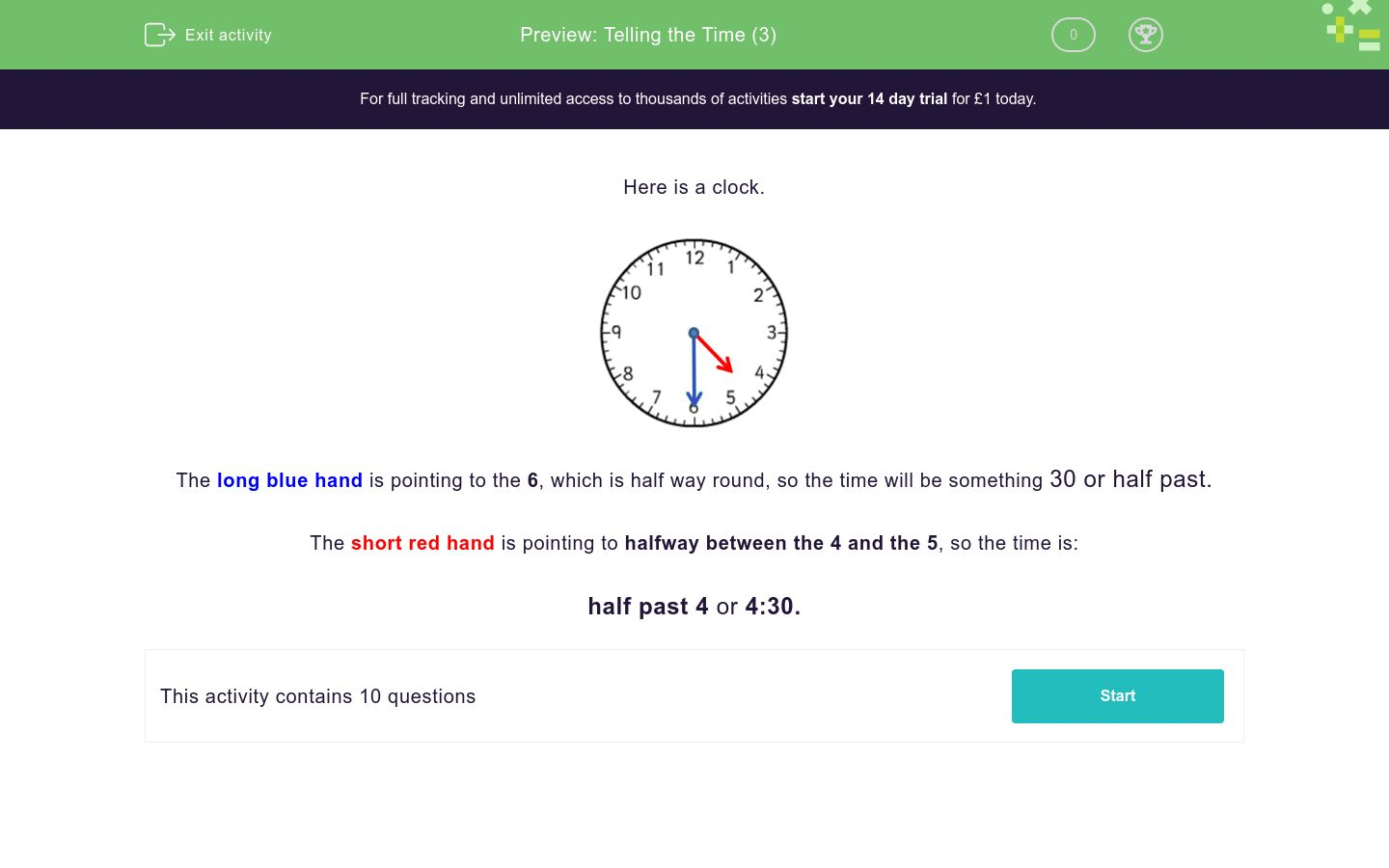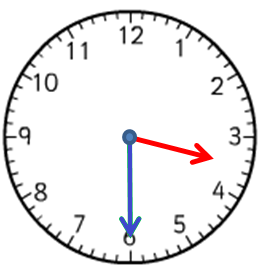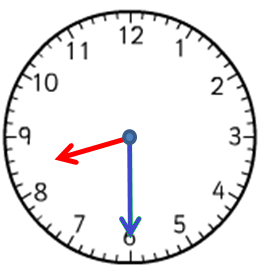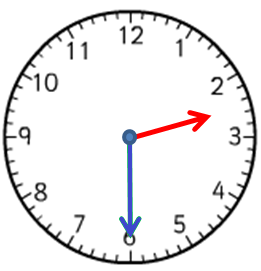# Telling the Time (3)

In this worksheet, students must select the time that matches the given clock face. Only half past times are shown.Key stage:  KS 1

Curriculum topic:   Measurement

Curriculum subtopic:   Tell the Time

Difficulty level:### QUESTION 1 of 10

Here is a clock.The long blue hand is pointing to the 6, which is half way round, so the time will be something 30 or half past.

The short red hand is pointing to halfway between the 4 and the 5, so the time is:

half past 4 or 4:30.

Which time is shown on the clock?7:30

6:30

8:30

Which time is shown on the clock?3 o'clock

6:20

3:30

Which time is shown on the clock?7:30

6:30

3:30

Write the time shown on the clock.

Use a colon : to separate the hour from the minutes (for example, 7:30).Write the time shown on the clock.

Use a colon : to separate the hour from the minutes (for example, 7:30).Write the time shown on the clock.

Use a colon : to separate the hour from the minutes (for example, 7:30).Write the time shown on the clock.

Use a colon : to separate the hour from the minutes (for example, 7:30).Write the time shown on the clock.

Use a colon : to separate the hour from the minutes (for example, 7:30).Which time is shown on the clock?half past 2

half past 3

half past 6

Which time is shown on the clock?half past 1

half past 6

half past 12

• Question 1

Which time is shown on the clock?7:30
EDDIE SAYS
The short hand is pointing to halfway between the 7 and the 8.
The long hand is pointing to 6.
• Question 2

Which time is shown on the clock?3:30
EDDIE SAYS
The short hand is pointing to halfway between the 3 and the 4.
The long hand is pointing to 6.
• Question 3

Which time is shown on the clock?6:30
EDDIE SAYS
The short hand is pointing to halfway between the 6 and the 7.
The long hand is pointing to 6.
• Question 4

Write the time shown on the clock.

Use a colon : to separate the hour from the minutes (for example, 7:30).11:30
EDDIE SAYS
The short hand is pointing to halfway between the 11 and the 12.
The long hand is pointing to 6.
• Question 5

Write the time shown on the clock.

Use a colon : to separate the hour from the minutes (for example, 7:30).05:30
5:30
EDDIE SAYS
The short hand is pointing to halfway between the 5 and the 6.
The long hand is pointing to 6.
• Question 6

Write the time shown on the clock.

Use a colon : to separate the hour from the minutes (for example, 7:30).08:30
8:30
EDDIE SAYS
The short hand is pointing to halfway between the 8 and the 9.
The long hand is pointing to 6.
• Question 7

Write the time shown on the clock.

Use a colon : to separate the hour from the minutes (for example, 7:30).01:30
1:30
EDDIE SAYS
The short hand is pointing to halfway between the 1 and the 2.
The long hand is pointing to 6.
• Question 8

Write the time shown on the clock.

Use a colon : to separate the hour from the minutes (for example, 7:30).10:30
EDDIE SAYS
The short hand is pointing to halfway between the 10 and the 11.
The long hand is pointing to 6.
• Question 9

Which time is shown on the clock?half past 2
EDDIE SAYS
The short hand is pointing to halfway between the 2 and the 3.
The long hand is pointing to 6.
• Question 10

Which time is shown on the clock?half past 12
EDDIE SAYS
The short hand is pointing to halfway between the 12 and the 1.
The long hand is pointing to 6.
---- OR ----

Sign up for a £1 trial so you can track and measure your child's progress on this activity.

### What is EdPlace?

We're your National Curriculum aligned online education content provider helping each child succeed in English, maths and science from year 1 to GCSE. With an EdPlace account you’ll be able to track and measure progress, helping each child achieve their best. We build confidence and attainment by personalising each child’s learning at a level that suits them.

Get started